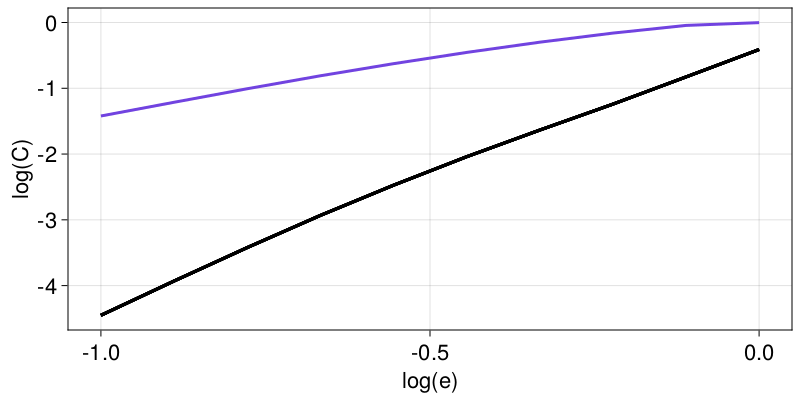# Multidimensional surrogates

Multidimensional surrogates operate typically on input StateSpaceSets and output the same type.

## Shuffle dimensions

This surrogate was made to distinguish multidimensional data with structure in the state space from multidimensional noise.

Here is a simple application that shows that the distinction is successful for a system that we know a-priori is deterministic and has structure in the state space (a chaotic attractor).

using TimeseriesSurrogates
using DynamicalSystemsBase
using FractalDimensions: correlationsum
using CairoMakie

# Create a trajectory from the towel map
function towel_rule(x, p, n)
@inbounds x1, x2, x3 = x, x, x
SVector( 3.8*x1*(1-x1) - 0.05*(x2+0.35)*(1-2*x3),
0.1*( (x2+0.35)*(1-2*x3) - 1 )*(1 - 1.9*x1),
3.78*x3*(1-x3)+0.2*x2 )
end
to = DeterministicIteratedMap(towel_rule, [0.1, -0.1, 0.1])
X = trajectory(to, 10_000; Ttr = 100)

e = 10.0 .^ range(-1, 0; length = 10)
CX = correlationsum(X, e; w = 5)

le = log10.(e)
fig, ax = lines(le, log10.(CX))

sg = surrogenerator(X, ShuffleDimensions())
for i in 1:10
Z = sg()
CZ = correlationsum(Z, e)
lines!(ax, le, log.(CZ); color = ("black", 0.8))
end
ax.xlabel = "log(e)"; ax.ylabel = "log(C)"
fig